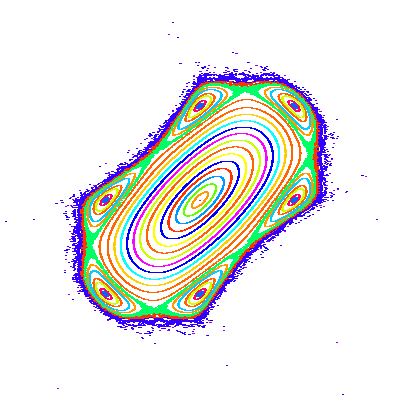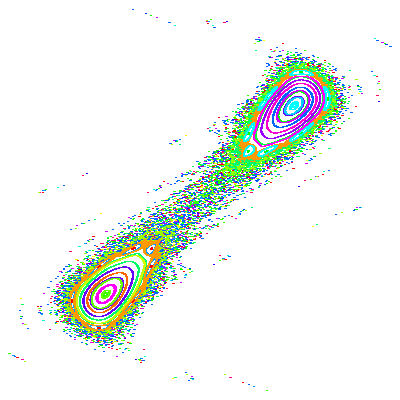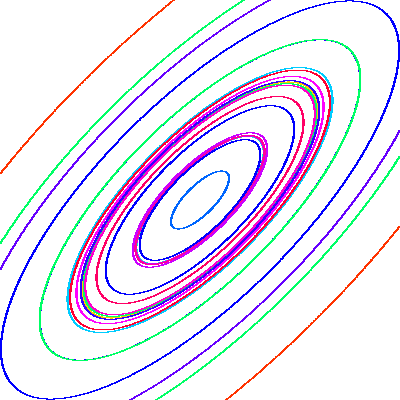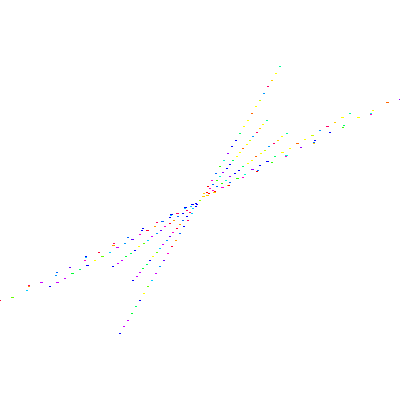Fall 2003

# Mathematics Math21b Fall 2003

## Linear Algebra and Differential Equations

Office: SciCtr 434
Email: knill@math.harvard.edu# The Cubic Henon Map

(An example of a nonlinear discrete dynamical system)
The cubic Henon map
 T(x,y) = ( g x-x3-y,x)
is a discrete dynamical system in the plane. The map is parameterized by a constant g. Below you see some orbits of the map for two different values of g. The point (0,0) is always an equilibrium point. As in the case of linear dynamical systems, one is interested about the stability of this fixed point. One can ask for example, which points stay for every in a bounded region and do not escap to infinity. In the picture to the left, you clearly see a large island around (0,0) which seems to stay, while in the picture to the right, a point starting near (0,0) escapes to infinity in general. Evenso this stability question is difficult, linear algebra helps to understand the system near the equilibrium point. For small x, the linear approximation of the map
 T(x,y) = ( g x-y,x) = A(x,y)
gives trajectories close to the real trajectories. We can analyse this linear system as we have learned: because the determinant D is 1 and the trace is g, the eigenvalues are g/2-(g2/4-1)1/2 and g/2-(g2/4-1)1/2. For g>2 there are two real eigenvalues, one smaller than 1, one bigger than 1 and the trajectories ly on hyperbola, and orbits escape to infinity. One can show that the nonlinear system also has curves invariant which ressemble the hyperbola in the linear case. For |g| smaller than 2, there are two complex eigenvalues of length 1. The linear system has then trajectories, which ly on ellipses. One can show using a theory called KAM that also the nonlinear system has curves invariant near the equilibrium point.The nonlinear system for g=1.5 The nonlinear system for g=2.5The linear system for g=1.5 The linear system for g=2.5

Back to the main page